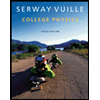# A 7.8 kg object experiences an acceleration of 1.3 m/s2. (a) What is the magnitude of the resultant force acting on it (in N)? N (b) If this same force is applied to a 4.7 kg object, what is the magnitude of the acceleration (in m/s2)? m/s2

Question
A 7.8 kg object experiences an acceleration of 1.3 m/s2.
(a)
What is the magnitude of the resultant force acting on it (in N)?
N
(b)
If this same force is applied to a 4.7 kg object, what is the magnitude of the acceleration (in m/s2)?
m/s2
Expert Solution

### Want to see the full answer?

Check out a sample Q&A hereStudents who’ve seen this question also like:College Physics
10th Edition
ISBN: 9781285737027
Author: Raymond A. Serway, Chris Vuille
Publisher: Cengage Learning